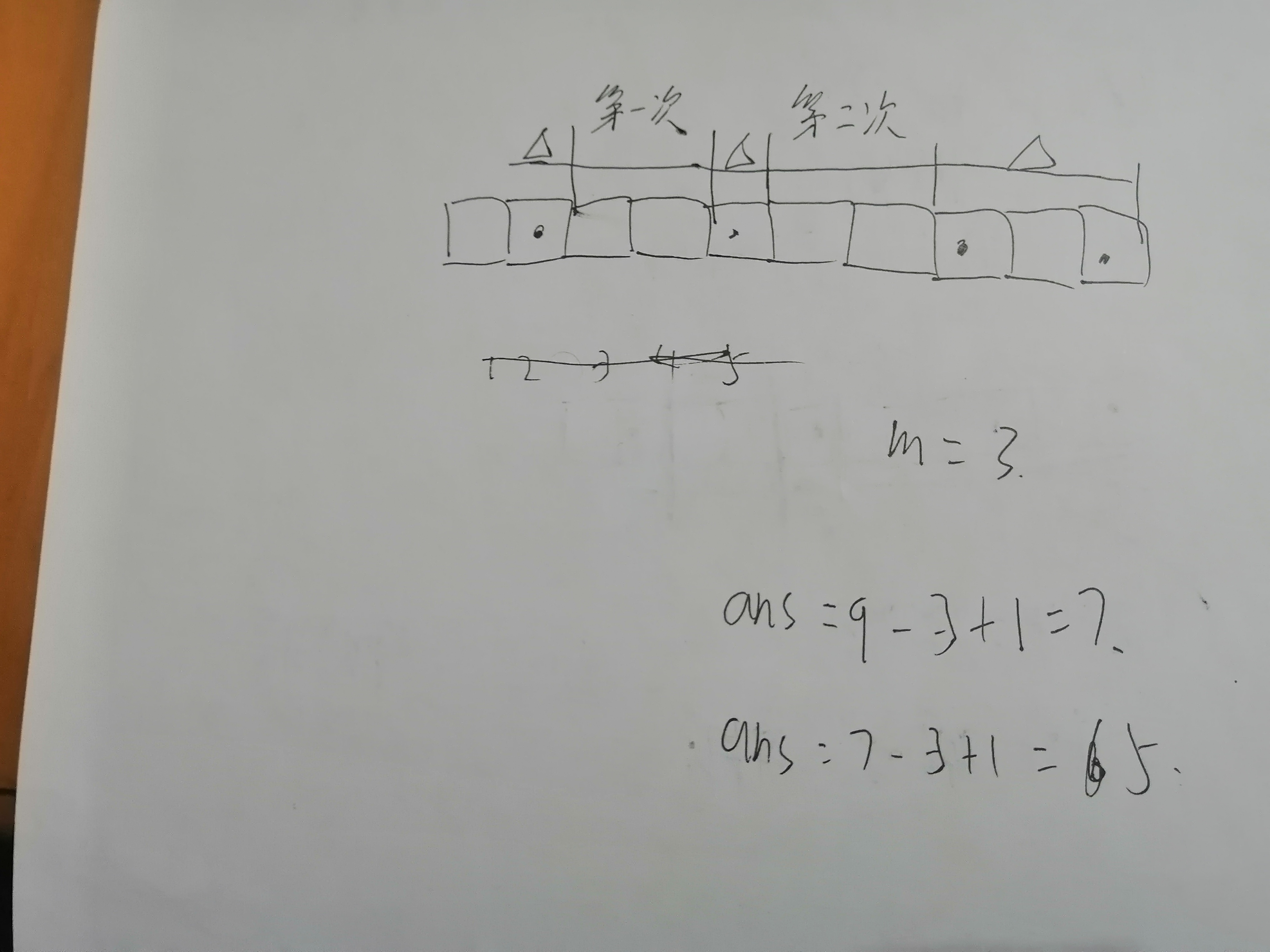# （区间相关问题）[USACO]修理牛棚 Barn Repair

## 一、算法分析## 二、代码及注释

#include<iostream>
#include<cstdio>
#include<cstring>
#include<vector>
#include<algorithm>
using namespace std;
vector<int> a;
vector<int> b;
bool cmp_new(int x1,int x2){
return x1>x2;
}
int main()
{

int m,s,c;
cin>>m>>s>>c;
if(m>=c){                         //注意这里有一个特判，当牛的数目小于最大木板数时，可以做到一牛一板
cout<<c;
return 0;
}
int x;
for(int i=0;i<c;i++){
cin>>x;
a.push_back(x);                  //a用来存牛所在的牛棚编号
}
sort(a.begin(),a.end());           //对编号排序（前面算法分析讲过）
for(int i=0;i<c-1;i++){
b.push_back(a[i+1]-a[i]-1);      //算出每个“空位”的长度
}
sort(b.begin(),b.end(),cmp_new);
int ans=a[c-1]-a+1;             //初始板长

for(int i=0;i<m-1;i++){            //截掉最大的m-1个空位
ans-=b[i];
}
cout<<ans;
return 0;
}


## 三、作者

Bowen

©️2019 CSDN 皮肤主题: 大白 设计师: CSDN官方博客